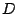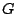# Perfect, Partitionable, and Kernel-Solvable Graphs

Given a graph G = (V,E), assign to every its edge e = (u,v) either the directed arc [u,v), or [v,u), or both. The obtained directed multi-graph D = (V,A) is called an orientation of G .

A vertex-subset K of V is called a KERNEL if K is (i) independent and (ii) absorbant, that is for each u from V K there is an arc [u,v) in A such that v in K.

Orientationis called clique acyclic if every clique ofhas a kernel in. Orientationis called kernel-less if it has no kernel.

Graph G is called kernel-solvable if every its clique-acyclic orientation has a kernel.

Berge and Duchet (1983) conjectured that (BD1) Perfect graphs are kernel-solvable, and (BD2) Kernel-solvable graphs are perfect.

BD1 was proved by Boros and Gurvich (1996) and by Holzman and Aharoni (1998), BD2 follows from the SPGC but no independent proof is known.

An orientation D of a PARTITIONABLE graph G is called UNIFORM if D is

(0) kernel-less and clique acyclic;

(a) for each maximum stable set S there exists a unique unabsorbed vertex v(S);

(b) v(S) belongs to the vis-a-vis clique C(S) of S;

(c) for each vertex v there exists a unique maximal stable set S(v) which does not absorb v .

Sebo (1998) proved that every kernel-less and clique-acyclic orientation of a minimal imperfect graph is uniform.

Conjecture. Each partitionable graph has a uniform orientation.

This, if true, implies BD2

Contributed by Boros and Gurvich

Back to the main index for Perfect Graphs.